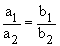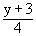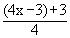Home MonkeyNotes Printable Notes Digital Library Study Guides Study Smart Parents Tips College Planning Test Prep Fun Zone Help / FAQ How to Cite New Title Request

1.5 Standard Forms For The Equation Of A Straight Line

1. A vertical line has equation x = a ( y-axis has equation
x = 0 ) , a Î R

2. A horizontal line has equation y = b (x-axis has equation y = 0), b Î RIf two straight lines are a1x + b1y + c1 = 0 and a2 x + b2y + c2 = 0

then¹for parallel lines

and (a1a2 + b1b2 = 0 )      for mutually perpendicular lines.

Example 1 If f : R ® R. f(x) = 4x-3x Î R. Find f -1, also find f o f -1 and f -1 o f.Your browser does not support the IFRAME tag.

Solution :

f : R ® R is one-one onto. Hence f -1 exists and f (x) = 4x - 3

Let y = f ( x ) = 4x-3 then 4x = y+3 \ x =\ f -1 : R ® R is given by f -1 ( y ) =or f -1 R ® R is given by f -1 (x) =(replacing y by x)

\ (f -1 o f ) : R ® R is given by

( f -1 o f ) ( x ) = f -1 [ f(x) ] = f -1 ( 4x-3 ) == x

and (f -1 o f) : R ® R is given by

( f o f -1 ) ( x ) = f [ f -1 (x) ] =Example 2

Let f : R ® R and g : R ® R and f (x) = x3 and g (x) = x2 + 1.

Find (1) f o f (2) g o g (3) g o f (4) f o g

Solution :

(1) ( f o f ) : R ® R is given as :

( f o f ) ( x ) = f [ f (x) ] = f [x3] = (x3) 3 = x9

Index

Introduction

1.1 Functions And Mapping
1.2 Functions, Their Graphs and Classification
1.3 Rules for Drawing the Graph of a Curve
1.4 Classification of Functions
1.5 Standard Forms for the equation of a straight line
1.6 Circular Function and Trigonometry

Chapter 2